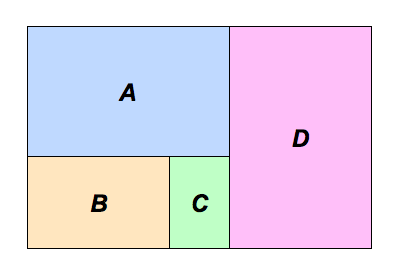# Five Similar Rectangles

The four rectangles $A$, $B$, $C$, and $D$ below, as well as the big rectangle they compose, are all similar to each other. The ratio of the area of $A$ to the area of $B$ can be written as $a:b$, where $a$ and $b$ are positive coprime integers. Give $a+b$.×